Hexapod Robot for the P1/P2 — Parallax Forums

# Hexapod Robot for the P1/P2

There are several hexapod robots out there but none of them are for the P1 or P2.

I did see the quick bite that controlled 16 servos so this project will need to control 18 servos. This winter project will actually make use of those servos, not just make them turn.

All the part will be 3D printed and require minimal hardware to put it together. I am a little concerned about the small servos sold by parallax. They have a working voltage of 3v to 4.8v. Why did they stop before 5 volts which is a very common voltage to use? Anyway, the servos that I will be using for this first go around will be some generic servos so all the part will be fitted for that purpose. My next build I will try to use the Micro bit servos.

This project will require floating point math as well as some geometry functions to calculate the position of all six legs. Inverse kinemetric.

So far, I have designed and printed the Coxa (hip), Femur, and Tibia (claw). In addition, I also printed a top and bottom shell to attach the legs to.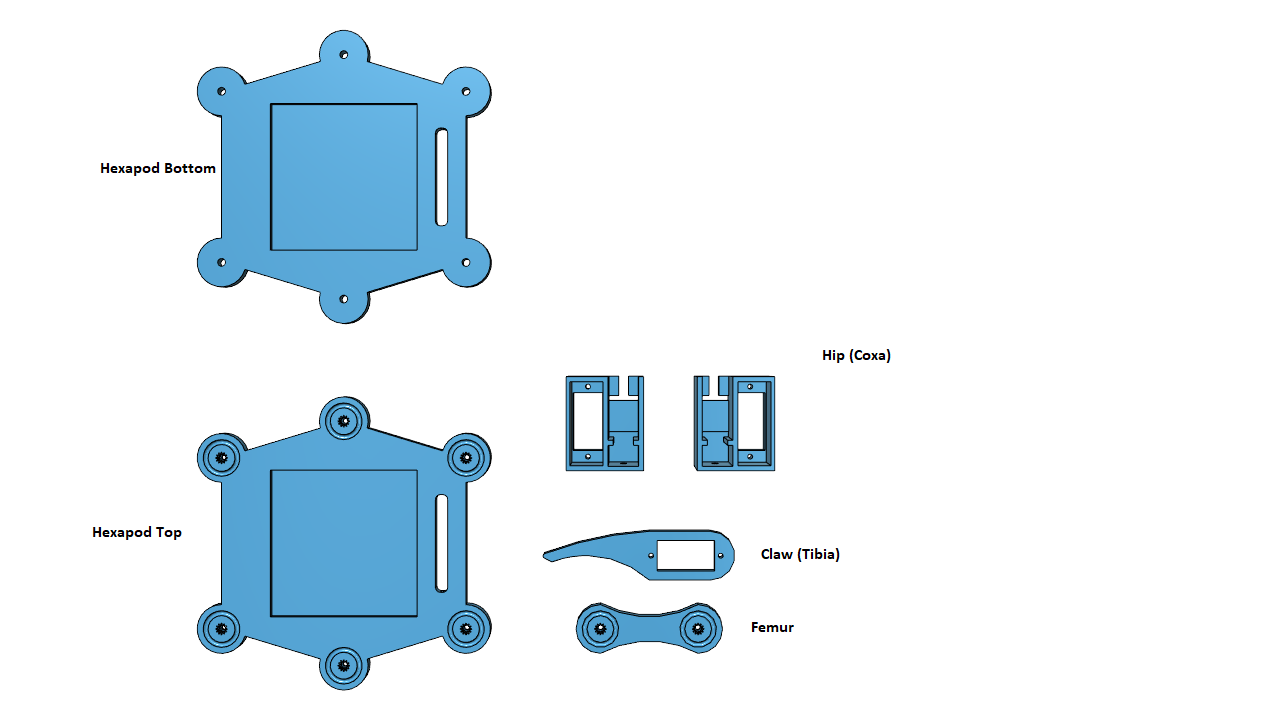One additional Item that I need is the servo controller. Either I use PCA9685 or I need to build a servo board to power the servos and run 18 wires for each servo.

Once the unit is assembled, I can set the servos to 90 degrees and start to calculate the x, y and z position of each leg.

I also plan to use an ESP8266 to send commands to the robot.

Mike

• I have not worked with these servos before so I thought I should check to see what PWM I need to send to get to 0, 90, and 180 degrees. Then I can develop a formula to convert angles into PWM values.

Here is the program I used to run the servo through its paces:

```#include <stdio.h>
#include <propeller.h>
#include "servos.h"
#include "HX8357.h"
#include "ina260.h"

#define LITE 14
#define RST 13
#define DC 12
#define CS 11
#define MOSI 10
#define MISO 9
#define CLK 8

#define INACLK 36

#define LEG1TIBIA 40
#define LEG1FEMUR 41
#define LEG1COXA 42

int i;
char Buffer;

int main(int argc, char** argv)
{

i = HX8357_open(CLK, MISO, MOSI, CS, DC, RST, LITE);

if ((i & 0x08) == 0)
HX8357_sleepOn(0);

// Is display on
if ((i & 0x04) == 0)
HX8357_displayOn(1);

i = HX8357_control();
printf("Control: %x\n", i);

HX8357_rotation(1);

HX8357_cls(HX8357_BLACK);

Servo_Enable(LEG1TIBIA, 1425, 20000);
Servo_Enable(LEG1FEMUR, 1500, 20000);
Servo_Enable(LEG1COXA, 1500, 20000);

INA260_setConfig(INA260_BOTH_CONTINUOUS, INA260_1100, INA260_1100, INA260_AVG1024, 0);

while (1)
{
_waitms(2000);
Servo_Set(LEG1TIBIA, 450);
_waitms(2000);
Servo_Set(LEG1TIBIA, 2400);
HX8357_writeStr(10, 10, "Voltage:           ");
i = INA260_getVoltage();
sprintf(Buffer, "%d", i);
HX8357_writeStr(150, 10, Buffer);
i = INA260_getCurrent();
sprintf(Buffer, "%d", i);
HX8357_writeStr(10, 50, "Current:           ");
HX8357_writeStr(150, 50, Buffer);
_waitms(2000);
Servo_Set(LEG1TIBIA, 1425);
}
}

// Angle 0 - 180 degrees
void setAngle(int angle)
{
int Range = 1950;

int pwm = 108*angle + 4500;
pwm = pwm / 10;
Servo_Set(LEG1TIBIA, pwm);
}
```

So, the range looks like 1950 or 10.8 pulses per degree. I used 10 times that and then divided it back out so I could avoid using floating point.

It's nice to be able to plug all this in and have it work. It took a while to solder the servo driver board and its only half done at this point.
Here is a video of the servo and rig in action:
Servo Rig

Mike

• It's been a while and I have been banging my head to see how to calculate the position of the leg knowing the angle of the coxa, femur and tibia. With the Scara I built before there were only two angles to consider and the Z axis was just the height you set. This is not the case with a Hexapod leg as it is moving in 3 dimensions at the same time.

The legs are attached along the body of the Hexapod at known points, so this is easy enough to figure out. This leave 6 calculations for each of the legs. The calculations are to determine from the center of the Hexapod where each leg will land in the X and Y plain. In addition, we want to know how high or low the leg is in the Y plain.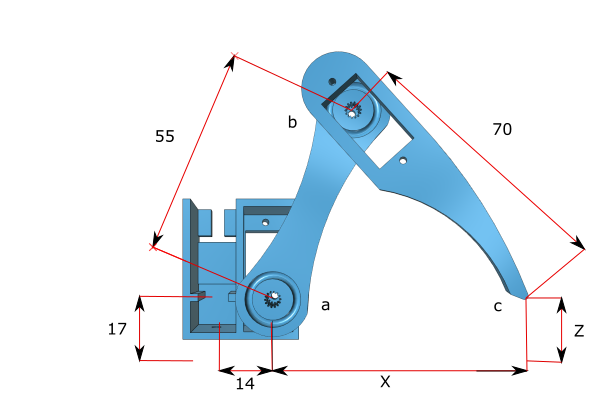From the above drawing there are a few things we know to help us figure out the value of X and Z. One of the problems is that each leg is mounted at an angle from the center of the Hexapod so that 180 degrees can be made use of with moving the servos around. This later will become and offset value.

One more thing is that looking down at the servos they turn counterclockwise from 0 degrees to 180 degrees. Since the servos are mounted to the top plate of the Hexapod they turn backwards so the angle will have to be subtracted from 180 to reverse the movement of the servo. The other two servos on the right side turn in the correct direction but the servos on the left side need to be reversed as well.

The legs are numbered from 1 to 6 in a counter clockwise fashion. The first servo is in the upper right corner and the 6th servo in the upper left corner.

Now to calculate X and Z knowing that the servo at a is 23 degrees from the Z axis and the servo at b is 42 degrees. Now if the angle at b was 90 degrees the distance between a and c would be the square root of a squared plus b squared. That only happens once in 180 degrees so that can’t be used.

We can however use the two right triangles that make up the femur and the tibia to get the distance of X.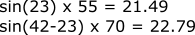So the X distance is 44.28. Now we need the Z distance which is the difference between the a height and the c height using the other side of the two right triangles.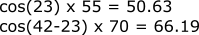That would make the difference -15.56 plus the height of the unit which is 17, so the leg is almost touching the ground.
Now we need to put it all together to find the X, Y, and Z point of the leg. The coxa is at 45 degrees making the final values as follows: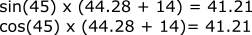If this was leg 1 then it would be located at X=76.21 (41.21 + 35) and Y=91.21 (41.21 + 50).

```Leg 2 is at (108.28, 0)
Leg 3 is at (76.21, -91.21)
Leg 4 is at (-76.21, -91.21)
Leg 5 is at (-108.28, 0)
Leg 6 is at (-76.21, 91.21)
```

This is all good but now I need to make the hexapod move and this is done by giving each leg a new location from where it is now. To do this we need to be able to determine the three angles based on the given X, Y, and Z point.

Leg 1 is located at (76.21, 91.21, 1.44) what angles make up this position:The coxa angle is 45 degrees and using the law of cosines I can determine the tibia angle: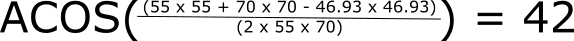I can use the same formula to get the femur angle: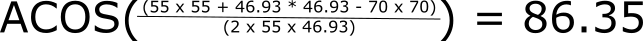From this angle we need to subtract 90 degrees to move it from the Y plain to the X plain. Now this angle is the angle of the femur-tibia and not associated with the X access. To get that angle we need to subtract the angle made by the X access and Z. We will use the arc tangent so that we get the proper sign of the angle so that we add or subtract that angle value to get the true femur angle.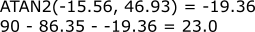So the femur angle is 23 degrees.
Using these two methods I can now determine where a leg is and how to place a leg.
Next step is write an interface program so that I can talk to the hexapod and tell it where to place the legs so that I can do calibrations. With attaching the servos to the legs the resulting angle is not correct and I will need to adjust those angles so that the leg will be at the point that is calculated. In addition the hexapod needs to have all the servos at the same angle so that when putting the leg together the legs are at the correct starting point. Having all the servos at 90 degrees, which is the mid point of the servos angle, it is easy enough to see if the joints are at that angle for assemble. In addition any servo can be used for any joint so assemble is straight forward.

• After some snafu's I got the Hexapod assembled.

I decided to update my servo library to include the servo angle function as well as being able to set the servo range. This interduce a bug that required several iterations to find it. It led me down several roads before I backed up and found it.

Anyway I now have the Parallax ESP8266 working with my control program written in C#. I'm able to use a pull down and have it find the ESP8266 unit and then make a connection to it so I can send commands. Right now I only have 3 commands: Get current angle of a leg, Move a leg or legs to an angle, and Move a leg to an X/Y position.

So using visual code to write the program for the P2 and using visual studio to write the control program worked out nicely. I had to account for servos on the left moving counterclockwise and also account for errors in assembly that led to an adjustment value for all the angles.

To communicate with the Hexapod I connect up to the telnet port on the ESP8266 and send it JSON objects which are broken down and then a JSON object is return to the Hexapod control program. At first things were not working until I found out that the C# program was sorting the JSON object fields. This led to a dilemma of either interpreting the object that way or finding another way to encode the JSON object so the fields were in the correct order.

I was able to find another way to encode the data so that the fields were in the correct order and all is good. One of the nice things is that I can have the visual code connected to the Hexapod at the same time the visual studio control program is connected. That way I can see the raw JSON data coming back from the Hexapod.

As you will see from the video fitting all that wire into the cavity of the Hexapod was a little bit of a challenge. I still need to find room for the battery.

Here is a capture of the Hexapod control program screen: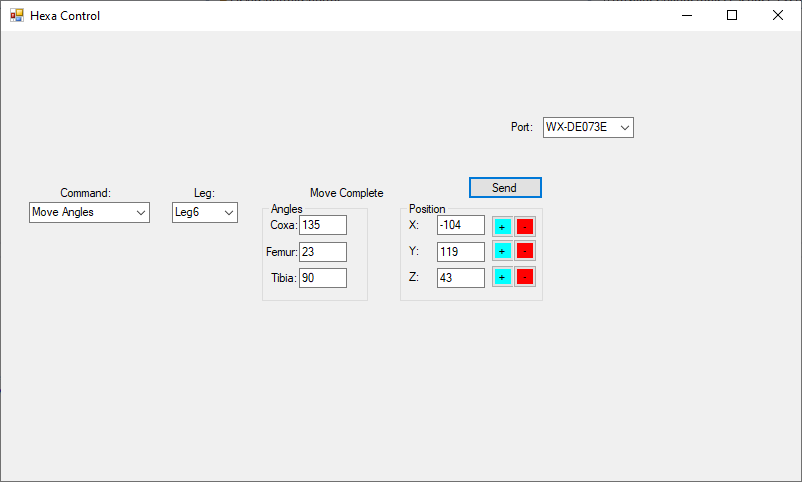The P2 Hexapod firmware:

```#include <stdio.h>
#include <propeller.h>
#include <math.h>
#include <libsimpletext/simpletext.h>
#include "json.h"
#include "servos.h"

void InitLegs(void);
void MoveLeg(int);
void SetLeg(int, int, int, int);
void DoServos(void *);
void SetResponse(int, int);
void DoCmd(int);
void GetAngles(void);
void MoveAngles(void);
void MovePoints(void);

void CalculateAngles(float x, float y, float z, float *, float *, float *);
void CalculatePoints(float, float, float, float *, float *, float *);
float LawOfCosine(float, float, float);
float LawOfCosineA(float, float, float);

#define LEG1TIBIA 21
#define LEG1FEMUR 22
#define LEG1COXA 23
#define LEG2TIBIA 11
#define LEG2FEMUR 12
#define LEG2COXA 13
#define LEG3TIBIA 8
#define LEG3FEMUR 9
#define LEG3COXA 10
#define LEG4TIBIA 40
#define LEG4FEMUR 41
#define LEG4COXA 42
#define LEG5TIBIA 43
#define LEG5FEMUR 44
#define LEG5COXA 45
#define LEG6TIBIA 46
#define LEG6FEMUR 56
#define LEG6COXA 57

struct Hexa
{
float Width;
float Length;
float Coxa;
float Femur;
float Tibia;
float Height;
} Hexapod = {.Width = 35, .Length = 50, .Coxa = 12.5, .Femur = 55, .Tibia = 70, .Height = 20};

/**
* 6 \   / 1
* 5 - x - 2
* 4 /   \ 3
*/
struct Leg
{
int coxa_pin; // coxa pin
int coxa; // coxa angle
int femur_pin; // femur pin
int femur; // femur angle
int tibia_pin; // tibia pin
int tibia; // tibia angle
int x; // x offset
int y; // y offset
int angle; // angle offset
} Legs;

struct info
{
int leg;
int x;
int y;
int z;
int coxa;
int femur;
int tibia;
} Info;

int Stack;
char Buffer;
char *ptr;

int main(int argc, char** argv)
{
int i;

_waitms(2000);

InitLegs();

i = 0;
while (1)
{
getStr(Buffer, 250);
json_init(Buffer);
ptr = json_find("cmd");
i = atoi(ptr);
DoCmd(i);
printf("\n");
}
}

void DoCmd(int cmd)
{
switch (cmd)
{
case 0: GetAngles();
break;
case 1: MoveAngles();
break;
case 2: MovePoints();
break;
}
}

void SetResponse(int r, int l)
{
float x, y, z;
float coxa, femur, tibia;

if (l > 5)
l = 0;
coxa = Legs[l].coxa;
femur = Legs[l].femur;
tibia = Legs[l].tibia;

CalculatePoints(coxa, femur, tibia, &x, &y, &z);
x = x + Legs[l].x;
y = y + Legs[l].y;
z = z + Hexapod.Height;

memset(Buffer, 0, sizeof(Buffer));
json_init(Buffer);
PutData("cmd", r);
PutData("leg", l);
PutData("x", x);
PutData("y", y);
PutData("z", z);
PutData("coxa", Legs[l].coxa);
PutData("femur", Legs[l].femur);
PutData("tibia", Legs[l].tibia);
printf(Buffer);
}

void PutData(char *item, int value)
{
char Data;

sprintf(Data, "%d", value);
json_putDec(item, Data);
}

void GetAngles()
{
int i;
float x, y, z;
float coxa, femur, tibia;

ptr = json_find("leg");
i = atoi(ptr);
SetResponse(128, i);
}

void MoveAngles()
{
int l;
int coxa, femur, tibia;

ptr = json_find("leg");
l = atoi(ptr);
if (l > 6)
return;
ptr = json_find("coxa");
coxa = atoi(ptr);
ptr = json_find("femur");
femur = atoi(ptr);
ptr = json_find("tibia");
tibia = atoi(ptr);
if (l == 6)
for (int i=0;i<6;i++)
SetLeg(i, coxa + Legs[i].angle, femur, tibia);
else
SetLeg(l, coxa, femur, tibia);
SetResponse(129, l);
}

void MovePoints()
{
int l;
int x, y, z;
float coxa, femur, tibia;

ptr = json_find("leg");
l = atoi(ptr);
if (l > 5)
return;
ptr = json_find("x");
x = atoi(ptr);
x = x - Legs[l].x;
ptr = json_find("y");
y = atoi(ptr);
y = y - Legs[l].y;
ptr = json_find("z");
z = atoi(ptr);
z = z - Hexapod.Height;

CalculateAngles(x, y, z, &coxa, &femur, &tibia);
SetLeg(l, coxa, femur, tibia);
SetResponse(130, l);
}

void InitLegs()
{
Legs.coxa_pin = LEG1COXA;
Legs.coxa = 45;
Legs.femur_pin = LEG1FEMUR;
Legs.femur = 90;
Legs.tibia_pin = LEG1TIBIA;
Legs.tibia = 90;
Legs.x = 35;
Legs.y = 50;
Legs.angle = -45;
Legs.coxa_pin = LEG2COXA;
Legs.coxa = 0;
Legs.femur_pin = LEG2FEMUR;
Legs.femur = 90;
Legs.tibia_pin = LEG2TIBIA;
Legs.tibia = 90;
Legs.x = 50;
Legs.y = 0;
Legs.angle = -90;
Legs.coxa_pin = LEG3COXA;
Legs.coxa = 315;
Legs.femur_pin = LEG3FEMUR;
Legs.femur = 90;
Legs.tibia_pin = LEG3TIBIA;
Legs.tibia = 90;
Legs.x = 35;
Legs.y = -50;
Legs.angle = 225;
Legs.coxa_pin = LEG4COXA;
Legs.coxa = 225;
Legs.femur_pin = LEG4FEMUR;
Legs.femur = 90;
Legs.tibia_pin = LEG4TIBIA;
Legs.tibia = 90;
Legs.x = -35;
Legs.y = -50;
Legs.angle = 135;
Legs.coxa_pin = LEG5COXA;
Legs.coxa = 180;
Legs.femur_pin = LEG5FEMUR;
Legs.femur = 90;
Legs.tibia_pin = LEG5TIBIA;
Legs.tibia = 90;
Legs.x = -50;
Legs.y = 0;
Legs.angle = 90;
Legs.coxa_pin = LEG6COXA;
Legs.coxa = 135;
Legs.femur_pin = LEG6FEMUR;
Legs.femur = 90;
Legs.tibia_pin = LEG6TIBIA;
Legs.tibia = 90;
Legs.x = -35;
Legs.y = 50;
Legs.angle = 45;

Servo_SetHighLow(2500, 450);

cogstart(DoServos, NULL, Stack, 50);
}

void DoServos(void *ptr)
{
for (int i=0;i<6;i++)
{
Servo_Enable(Legs[i].coxa_pin, 0, 20000);
Servo_Enable(Legs[i].femur_pin, 0, 20000);
Servo_Enable(Legs[i].tibia_pin, 0, 20000);
}

while (1)
{
for (int i=0;i<6;i++)
MoveLeg(i);
_waitms(500);
}

}

void MoveLeg(int leg)
{
_waitms(50);
int c = Legs[leg].coxa - Legs[leg].angle;
c = 180 - c + Legs[leg].coxa_adj;
if (leg > 2)
{
Servo_SetAngle(Legs[leg].coxa_pin, c);
Servo_SetAngle(Legs[leg].femur_pin, 180 - Legs[leg].femur - Legs[leg].femur_adj);
Servo_SetAngle(Legs[leg].tibia_pin, 180 - Legs[leg].tibia - Legs[leg].tibia_adj);
}
else
{
Servo_SetAngle(Legs[leg].coxa_pin, c);
}
}

void SetLeg(int leg, int coxa, int femur, int tibia)
{
Legs[leg].coxa = coxa;
Legs[leg].femur = femur;
Legs[leg].tibia = tibia;
}

void CalculateAngles(float x, float y, float z, float *coxa, float *femur, float *tibia)
{
float CA = atan2(y, x) * 180 / PI;
if (CA < 0)
CA = CA + 360;
*coxa = CA;

float TR = sqrt(x * x + y * y);
TR = TR - Hexapod.Coxa;

float TC = sqrt(TR * TR + z * z);
*tibia = LawOfCosineA(Hexapod.Tibia, Hexapod.Femur, TC);
float FA = LawOfCosineA(TC, Hexapod.Femur, Hexapod.Tibia);
*femur = atan2(z, TR) * 180 / PI;
*femur = 90 - FA - *femur;
}

void CalculatePoints(float coxa, float femur, float tibia, float *x, float *y, float *z)
{
coxa = coxa * PI / 180;
femur = femur * PI / 180;
tibia = tibia * PI / 180;

float X = Hexapod.Coxa + Hexapod.Femur * sin(femur) + Hexapod.Tibia * sin(tibia - femur);
float Z = Hexapod.Femur * cos(femur) - Hexapod.Tibia * cos(tibia - femur);
*x = X * cos(coxa);
*y = X * sin(coxa);
*z = Z;
}

/**
* Given two sides and angle c return the length of side c
*/
float LawOfCosine(float a, float b, float anglec)
{
anglec = anglec * PI / 180;
float v = sqrt(a * a + b * b - 2 * a * b * cos(anglec));

return v;
}

/**
* Given three side return the angle of side c
*/
float LawOfCosineA(float a, float b, float sidec)
{
float v = (a * a + b * b - sidec * sidec) / (2 * a * b);

if (v > 1.0f)
return 0;
if (v < -1.0f)
return 180;

return acos(v) * 180 / PI;
}
```

Sample of the JSON data:

```{"cmd":129, "leg":0, "x":75, "y":90, "z":4, "coxa":45, "femur":23, "tibia":42}
{"cmd":128, "leg":0, "x":75, "y":90, "z":4, "coxa":45, "femur":23, "tibia":42}
{"cmd":130, "leg":0, "x":74, "y":89, "z":-9, "coxa":45, "femur":43, "tibia":48}
{"cmd":129, "leg":0, "x":74, "y":89, "z":-9, "coxa":45, "femur":43, "tibia":48}
{"cmd":128, "leg":1, "x":106, "y":0, "z":-9, "coxa":0, "femur":43, "tibia":48}
{"cmd":130, "leg":1, "x":149, "y":0, "z":49, "coxa":0, "femur":22, "tibia":94}
```

Video of the Hexapod in action:
Next step is to make it walk.

Mike

Hexapod Parts

• edited 2022-01-14 13:20

Success, The Hexapod is walking using Gate1.

Gate1 is where three legs are lifted while the other 3 hold up the hexapod. Then move the up legs forward and the down legs backwards. From there you put the three legs down and repeat the process. To move the exact distance, you would divide the distance by 2.

I had to update the code so that I could tell when a movement was complete before moving to the next. A new function was added call move hexapod.

Updated code with move hexapod added:

```#include <stdio.h>
#include <propeller.h>
#include <math.h>
#include <stdbool.h>
#include <libsimpletext/simpletext.h>
#include "json.h"
#include "servos.h"

void InitLegs(void);
void MoveLeg(int);
int GetDiff(int, int);
bool CheckBusy(void);
void SetLeg(int, int, int, int);
void DoServos(void *);
void SetResponse(int, int);
void DoCmd(int);
void GetAngles(void);
void MoveAngles(void);
void MovePoints(void);
void MoveIt(void);
void Gate1(int, int, int);
void MoveHexapod(int, int, int, int);

void CalculateAngles(float x, float y, float z, float *, float *, float *);
void CalculatePoints(float, float, float, float *, float *, float *);
float LawOfCosine(float, float, float);
float LawOfCosineA(float, float, float);

#define LEG1TIBIA 21
#define LEG1FEMUR 22
#define LEG1COXA 23
#define LEG2TIBIA 11
#define LEG2FEMUR 12
#define LEG2COXA 13
#define LEG3TIBIA 8
#define LEG3FEMUR 9
#define LEG3COXA 10
#define LEG4TIBIA 40
#define LEG4FEMUR 41
#define LEG4COXA 42
#define LEG5TIBIA 43
#define LEG5FEMUR 44
#define LEG5COXA 45
#define LEG6TIBIA 46
#define LEG6FEMUR 56
#define LEG6COXA 57

struct Hexa
{
float Width;
float Length;
float Coxa;
float Femur;
float Tibia;
float Height;
} Hexapod = {.Width = 35, .Length = 50, .Coxa = 12.5, .Femur = 55, .Tibia = 70, .Height = 20};

/**
* 6 \   / 1
* 5 - x - 2
* 4 /   \ 3
*/
struct Leg
{
int coxa_pin; // coxa pin
int coxa; // coxa angle
int femur_pin; // femur pin
int femur; // femur angle
int tibia_pin; // tibia pin
int tibia; // tibia angle
int x; // x offset
int y; // y offset
int angle; // angle offset
bool busy; // leg is moving
} Legs;

struct mov
{
int coxa;
int femur;
int tibia;
} Mov;

struct info
{
int leg;
int x;
int y;
int z;
int coxa;
int femur;
int tibia;
} Info;

int Stack;
char Buffer;
char *ptr;

int main(int argc, char** argv)
{
int i;

_waitms(2000);

printf("Initilizing\n");

InitLegs();

i = 0;
while (1)
{
getStr(Buffer, 250);
json_init(Buffer);
ptr = json_find("cmd");
i = atoi(ptr);
DoCmd(i);
printf("\n");
}
}

void DoCmd(int cmd)
{
switch (cmd)
{
case 0: GetAngles();
break;
case 1: MoveAngles();
break;
case 2: MovePoints();
break;
case 3: MoveIt();
break;
}
}

void SetResponse(int r, int l)
{
float x, y, z;
float coxa, femur, tibia;

if (l > 5)
l = 0;

while (Legs[l].busy)
_waitms(100);

coxa = Mov[l].coxa;
femur = Mov[l].femur;
tibia = Mov[l].tibia;

CalculatePoints(coxa, femur, tibia, &x, &y, &z);
x = x + Legs[l].x;
y = y + Legs[l].y;
z = z + Hexapod.Height;

memset(Buffer, 0, sizeof(Buffer));
json_init(Buffer);
PutData("cmd", r);
PutData("leg", l);
PutData("x", x);
PutData("y", y);
PutData("z", z);
PutData("coxa", Mov[l].coxa);
PutData("femur", Mov[l].femur);
PutData("tibia", Mov[l].tibia);
printf(Buffer);
}

void PutData(char *item, int value)
{
char Data;

sprintf(Data, "%d", value);
json_putDec(item, Data);
}

void GetAngles()
{
int i;
float x, y, z;
float coxa, femur, tibia;

ptr = json_find("leg");
i = atoi(ptr);
SetResponse(128, i);
}

void MoveAngles()
{
int l;
int coxa, femur, tibia;

ptr = json_find("leg");
l = atoi(ptr);
if (l > 6)
return;
ptr = json_find("coxa");
coxa = atoi(ptr);
ptr = json_find("femur");
femur = atoi(ptr);
ptr = json_find("tibia");
tibia = atoi(ptr);
if (l == 6)
for (int i=0;i<6;i++)
SetLeg(i, coxa - Legs[i].angle, femur, tibia);
else
SetLeg(l, coxa, femur, tibia);
SetResponse(129, l);
}

void MovePoints()
{
int leg;
int x, y, z;
float coxa, femur, tibia;

ptr = json_find("leg");
leg = atoi(ptr);
if (leg > 5)
return;
ptr = json_find("x");
x = atoi(ptr);
x = x - Legs[leg].x;
ptr = json_find("y");
y = atoi(ptr);
y = y - Legs[leg].y;
ptr = json_find("z");
z = atoi(ptr);
z = z - Hexapod.Height;

CalculateAngles(x, y, z, &coxa, &femur, &tibia);
if (coxa > -Legs[leg].angle + 180)
coxa -= 360;
if (coxa < -Legs[leg].angle)
coxa += 360;

SetLeg(leg, coxa, femur, tibia);
SetResponse(130, leg);
}

void MoveIt()
{
int l;
int x, y, z;
int x1, y1;

ptr = json_find("leg");
l = atoi(ptr);
ptr = json_find("x");
x = atoi(ptr);
ptr = json_find("y");
y = atoi(ptr);
ptr = json_find("z");
z = atoi(ptr);

while ((x != 0) || (y != 0))
{
x1 = x;
y1 = y;

if (x > 10)
x1 = 10;

if (x < -10)
x1 = -10;

if (y > 10)
y1 = 10;

if (y < -10)
y1 = -10;

Gate1(x1, y1, z);

if (x != 0)
x = x - x1;
if (y != 0)
y = y - y1;
}

SetResponse(131, 0);

}

void Gate1(int x, int y, int z)
{
int i;

// pickup even legs
for (i=0;i<6;i++)
if (i & 1)
{
MoveHexapod(i, 0, 0, z);
Legs[i].busy = true;
}

while (CheckBusy())
_waitms(100);

// first move
for (i=0;i<6;i++)
if (i & 1)
MoveHexapod(i, x, y, 0);
else
MoveHexapod(i, -x, -y, 0);

for (i=0;i<6;i++)
Legs[i].busy = true;

while (CheckBusy())
_waitms(100);

// put leg down
for (i=0;i<6;i++)
if (i & 1)
{
MoveHexapod(i, 0, 0, -z);
Legs[i].busy = true;
}

while (CheckBusy())
_waitms(100);

// pickup even leg
for (i=0;i<6;i++)
if ((i & 1) == 0)
{
MoveHexapod(i, 0, 0, z);
Legs[i].busy = true;
}

for (i=0;i<6;i++)
Legs[i].busy = true;

while (CheckBusy())
_waitms(100);

// second move
for (i=0;i<6;i++)
if (i & 1)
MoveHexapod(i, -x, -y, 0);
else
MoveHexapod(i, x, y, 0);

for (i=0;i<6;i++)
Legs[i].busy = true;

while (CheckBusy())
_waitms(100);

// put leg down
for (i=0;i<6;i++)
if ((i & 1) == 0)
{
MoveHexapod(i, 0, 0, -z);
Legs[i].busy = true;
}

while (CheckBusy())
_waitms(100);
}

void MoveHexapod(int leg, int x, int y, int z)
{
float coxa, femur, tibia;
float x1, y1, z1;

coxa = Legs[leg].coxa;
femur = Legs[leg].femur;
tibia = Legs[leg].tibia;
CalculatePoints(coxa, femur, tibia, &x1, &y1, &z1);
x1 = x1 + x;
y1 = y1 + y;
z1 = z1 + z;
CalculateAngles(x1, y1, z1, &coxa, &femur, &tibia);
// fix servo range for some angles
if (coxa > -Legs[leg].angle + 180)
coxa -= 360;
if (coxa < -Legs[leg].angle)
coxa += 360;
Mov[leg].coxa = coxa;
Mov[leg].femur = femur;
Mov[leg].tibia = tibia;
}

void InitLegs()
{
Legs.coxa_pin = LEG1COXA;
Legs.coxa = 45;
Legs.femur_pin = LEG1FEMUR;
Legs.femur = 90;
Legs.tibia_pin = LEG1TIBIA;
Legs.tibia = 90;
Legs.x = 35;
Legs.y = 50;
Legs.angle = 45;
Legs.busy = true;
Legs.coxa_pin = LEG2COXA;
Legs.coxa = 0;
Legs.femur_pin = LEG2FEMUR;
Legs.femur = 90;
Legs.tibia_pin = LEG2TIBIA;
Legs.tibia = 90;
Legs.x = 50;
Legs.y = 0;
Legs.angle = 90;
Legs.busy = true;
Legs.coxa_pin = LEG3COXA;
Legs.coxa = 315;
Legs.femur_pin = LEG3FEMUR;
Legs.femur = 90;
Legs.tibia_pin = LEG3TIBIA;
Legs.tibia = 90;
Legs.x = 35;
Legs.y = -50;
Legs.angle = -225;
Legs.busy = true;
Legs.coxa_pin = LEG4COXA;
Legs.coxa = 225;
Legs.femur_pin = LEG4FEMUR;
Legs.femur = 90;
Legs.tibia_pin = LEG4TIBIA;
Legs.tibia = 90;
Legs.x = -35;
Legs.y = -50;
Legs.angle = -135;
Legs.busy = true;
Legs.coxa_pin = LEG5COXA;
Legs.coxa = 180;
Legs.femur_pin = LEG5FEMUR;
Legs.femur = 90;
Legs.tibia_pin = LEG5TIBIA;
Legs.tibia = 90;
Legs.x = -50;
Legs.y = 0;
Legs.angle = -90;
Legs.busy = true;
Legs.coxa_pin = LEG6COXA;
Legs.coxa = 135;
Legs.femur_pin = LEG6FEMUR;
Legs.femur = 90;
Legs.tibia_pin = LEG6TIBIA;
Legs.tibia = 90;
Legs.x = -35;
Legs.y = 50;
Legs.angle = -45;
Legs.busy = true;

Servo_SetHighLow(2400, 450);

printf("Starting Servos\n");
cogstart(DoServos, NULL, Stack, 100);
}

void DoServos(void *ptr)
{

for (int i=0;i<6;i++)
{
Servo_Enable(Legs[i].coxa_pin, 0, 20000);
Servo_Enable(Legs[i].femur_pin, 0, 20000);
Servo_Enable(Legs[i].tibia_pin, 0, 20000);
Mov[i].coxa = Legs[i].coxa;
Mov[i].femur = Legs[i].femur;
Mov[i].tibia = Legs[i].tibia;
}

while (1)
{
for (int i=0;i<6;i++)
if (Legs[i].busy)
{
MoveLeg(i);
_waitms(20);
}
_waitms(50);
}

}

void MoveLeg(int leg)
{
int coxa, femur, tibia;
int diff;

diff = GetDiff(Legs[leg].coxa, Mov[leg].coxa);
Legs[leg].coxa = Legs[leg].coxa - diff;
diff = GetDiff(Legs[leg].femur, Mov[leg].femur);
Legs[leg].femur = Legs[leg].femur - diff;
diff = GetDiff(Legs[leg].tibia, Mov[leg].tibia);
Legs[leg].tibia = Legs[leg].tibia - diff;

coxa = Legs[leg].coxa + Legs[leg].angle + Legs[leg].coxa_adj;

if ((coxa < 0) || (coxa > 180))
printf("Coxa(%d): %d\n", leg+1, coxa);
if ((femur < 0) || (femur > 180))
printf("Femur(%d): %d\n", leg+1, femur);
if ((tibia < 0) || (tibia > 180))
printf("Tibia(%d): %d\n", leg+1, tibia);

if (leg > 2)
{
Servo_SetAngle(Legs[leg].coxa_pin, 180 - coxa);
Servo_SetAngle(Legs[leg].femur_pin, 180 - femur);
Servo_SetAngle(Legs[leg].tibia_pin, 180 - tibia);
}
else
{
Servo_SetAngle(Legs[leg].coxa_pin, 180 - coxa);
Servo_SetAngle(Legs[leg].femur_pin, femur);
Servo_SetAngle(Legs[leg].tibia_pin, tibia);
}

diff = abs(GetDiff(Legs[leg].coxa, Mov[leg].coxa));
diff += abs(GetDiff(Legs[leg].femur, Mov[leg].femur));
diff += abs(GetDiff(Legs[leg].tibia, Mov[leg].tibia));
if (diff == 0)
Legs[leg].busy = false;
}

int GetDiff(int angle1, int angle2)
{
int diff;

diff = angle1 - angle2;
if (diff > 10)
diff = 10;
if (diff < -10)
diff = -10;
return diff;
}

bool CheckBusy()
{
bool b;

b = false;
for (int i=0;i<6;i++)
if (Legs[i].busy == true)
b = true;

return b;
}

void SetLeg(int leg, int coxa, int femur, int tibia)
{
Mov[leg].coxa = coxa;
Mov[leg].femur = femur;
Mov[leg].tibia = tibia;
Legs[leg].busy = true;
}

void CalculateAngles(float x, float y, float z, float *coxa, float *femur, float *tibia)
{
float CA = atan2(y, x) * 180 / PI;
if (CA < 0)
CA = CA + 360;
*coxa = CA;

float TR = sqrt(x * x + y * y);
TR = TR - Hexapod.Coxa;

float TC = sqrt(TR * TR + z * z);
*tibia = LawOfCosineA(Hexapod.Tibia, Hexapod.Femur, TC);
float FA = LawOfCosineA(TC, Hexapod.Femur, Hexapod.Tibia);
*femur = atan2(z, TR) * 180 / PI;
*femur = 90 - FA - *femur;
}

void CalculatePoints(float coxa, float femur, float tibia, float *x, float *y, float *z)
{
coxa = coxa * PI / 180;
femur = femur * PI / 180;
tibia = tibia * PI / 180;

float X = Hexapod.Coxa + Hexapod.Femur * sin(femur) + Hexapod.Tibia * sin(tibia - femur);
float Z = Hexapod.Femur * cos(femur) - Hexapod.Tibia * cos(tibia - femur);
*x = X * cos(coxa);
*y = X * sin(coxa);
*z = Z;
}

/**
* Given two sides and angle c return the length of side c
*/
float LawOfCosine(float a, float b, float anglec)
{
anglec = anglec * PI / 180;
float v = sqrt(a * a + b * b - 2 * a * b * cos(anglec));

return v;
}

/**
* Given three side return the angle of side c
*/
float LawOfCosineA(float a, float b, float sidec)
{
float v = (a * a + b * b - sidec * sidec) / (2 * a * b);

if (v > 1.0f)
return 0;
if (v < -1.0f)
return 180;

return acos(v) * 180 / PI;
}
```

Mike